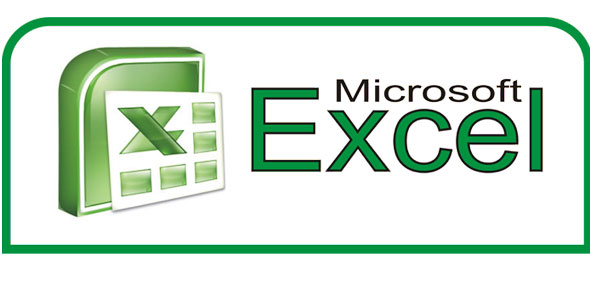# Microsoft Excel Formatting & Basic Formulas

6 Questions | Total Attempts: 149SettingsMicrosoft Excel 2007 Essentials Training

Related Topics
• 1.
What will be the output if you format the cell containing 5436.8 as '#,##0.00'
• A.

A. 6.8

• B.

B. 5,436.8

• C.

C. 5,436.80

• D.

D. 5,430.00

• 2.
What do you type into an empty cell to start a formula?
• A.

*

• B.

(

• C.

=

• D.

#

• 3.
"&" sign is commonly use to concatenate or join things together?
• A.

True

• B.

False

• 4.
Which area in an Excel window allows entering values and formulas?
• A.

A. Tittle Bar

• B.

• C.

C. Formula bar

• D.

D. Standard Tool Bar

• 5.
A value used in a formula that does not change is called a ________?
• A.

• B.

B. Constant

• C.

C. Variable

• D.

D. Static

• 6.
Is the result for "=A1+A2*A3" same with "=(A1+A2)*A3"?
• A.

True

• B.

False